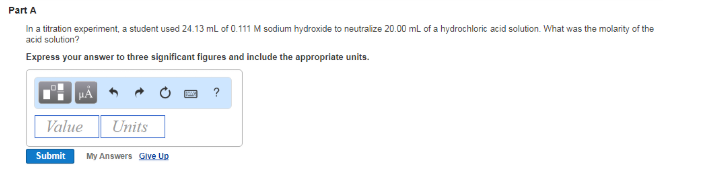Chemistry Practice Problems Equivalence Point Practice Problems Solution: In a titration experiment, a student used 24.13 mL...

# Solution: In a titration experiment, a student used 24.13 mL of 0.111 M sodium hydroxide to neutralize 20.00 mL of a hydrochloric acid solution. What was the molarity of the acid solution? Express your answer to three significant figures and include the appropriate units.

###### Problem

In a titration experiment, a student used 24.13 mL of 0.111 M sodium hydroxide to neutralize 20.00 mL of a hydrochloric acid solution. What was the molarity of the acid solution? Express your answer to three significant figures and include the appropriate units.View Complete Written Solution

Equivalence Point

Equivalence Point

#### Q. A volume of 80.0 mL of a 0.610 M HNO3 solution is titrated with 0.150 M KOH. Calculate the volume of KOH required to reach the equivalence point. Expr...

Solved • Tue Jul 17 2018 14:41:44 GMT-0400 (EDT)

Equivalence Point

#### Q. Calculate the volume, in milliliters, of a 0.19 M NaOH solution that will completely neutralize each of the following: Part A 30.0 mL of a 0.295 M HCl...

Solved • Tue Jul 17 2018 13:21:20 GMT-0400 (EDT)

Equivalence Point

#### Q. Part AWhat is the molarity of a solution of HCl if 6.00 mL of the HCl solution is titrated with 33.0 mL of a 0.170 M NaOH solution? HCl (aq) + NaOH (a...

Solved • Tue Jul 17 2018 13:18:45 GMT-0400 (EDT)

Equivalence Point

#### Q. What is the concentration of an HBr solution if 31.0 mL of a 0.200 M NaOH solution are needed to titrate 55.0 mL of the acid?

Solved • Tue Jul 17 2018 12:37:35 GMT-0400 (EDT)